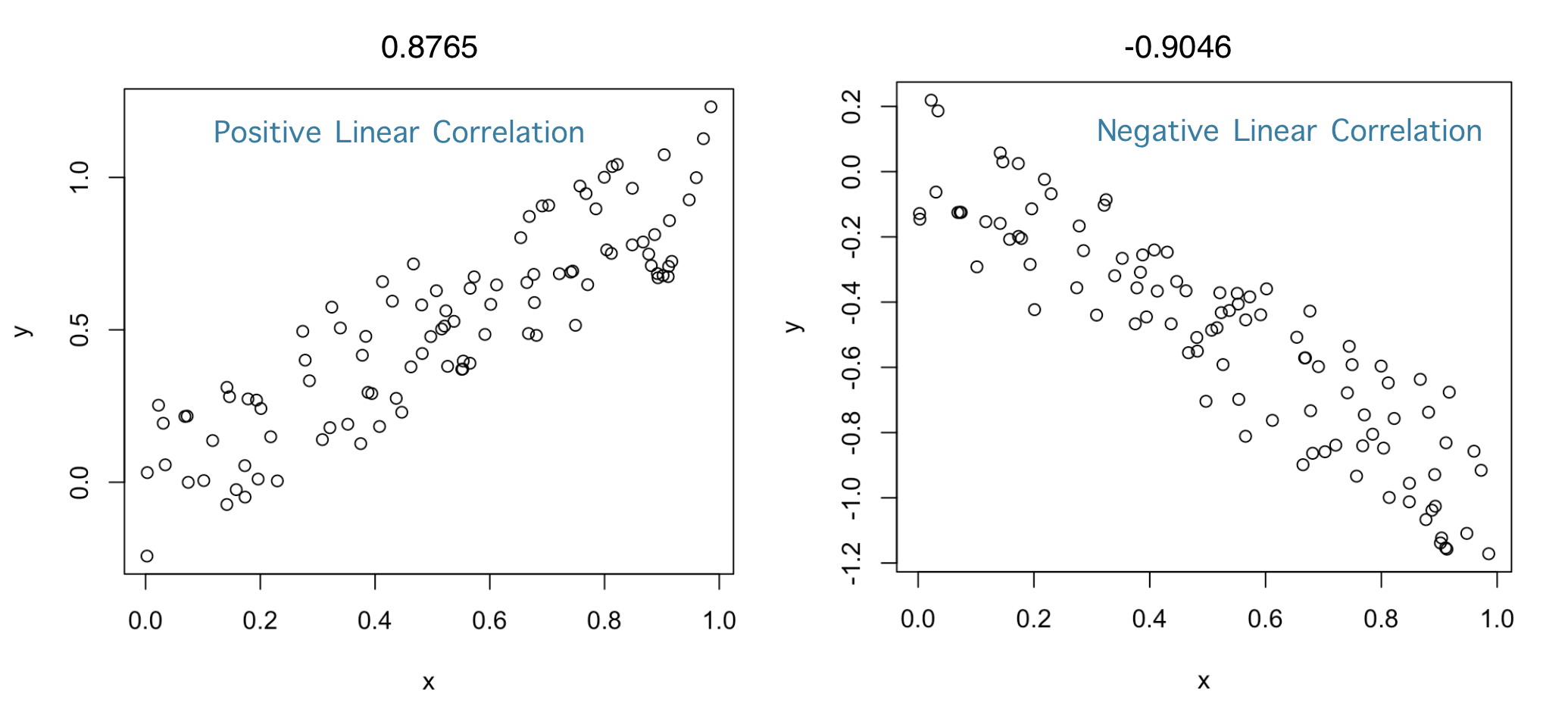### Some univariate statistics notation

• $$X$$ is a random variable
• In data: $$X_i$$ is the value of the variable for entry $$i$$, for example the GDP of a country
• $$E[X]$$ is the expected value of $$X$$
• We estimate the expected value as the mean of $$X$$: $$\mu_X = \frac{1}{N}\sum_i X_i$$
• $$N$$ is the number of data points, for example the number of countries
• In R you can calculate it with the function mean()
• $$V[X]$$ is the variance of $$X$$
• We calculate it as the expected squared difference to the mean X: $$V[X] = \frac{1}{N}\sum_i (X_i - \mu_X)^2$$
• The variance is measured in the square of units of X (e.g. if X is GDP measured in USD, then V[X] is USD$$^2$$)
• $$\sigma_X$$ is the standard deviation of X
• $$\sigma_X = \sqrt{V[X]}$$, which is convenient because it measures dispersion in the same units as $$X$$
• in R you can calculate it with the function sd()

### Pearson’s Correlation Coefficient

Correlation: Linear association or dependence between the values of variables X and Y

Pearson’s way to measure correlation between variables $$X$$ and $$Y$$, denoted as $$\rho(X,Y)$$:

• If X and Y are independent, they satisfy that the expectation of the product equals the product of expectations:

$$E[XY] = E[X]E[Y]$$

• The principle: measure correlation as the deviation from $$E[XY] − E [X]E[Y] = 0$$
• The absolute value of this difference can be at most $$\sigma_X\sigma_Y$$
• $$\rho(X,Y)$$ rescales the difference to be between −1 and 1

$$\rho(X,Y) = \frac{E[XY] − E [X]E[Y]}{\sigma_X\sigma_Y}$$

• Can be computed in R with the function cor()

### Some examples of Pearson’s Correlation Coefficient: